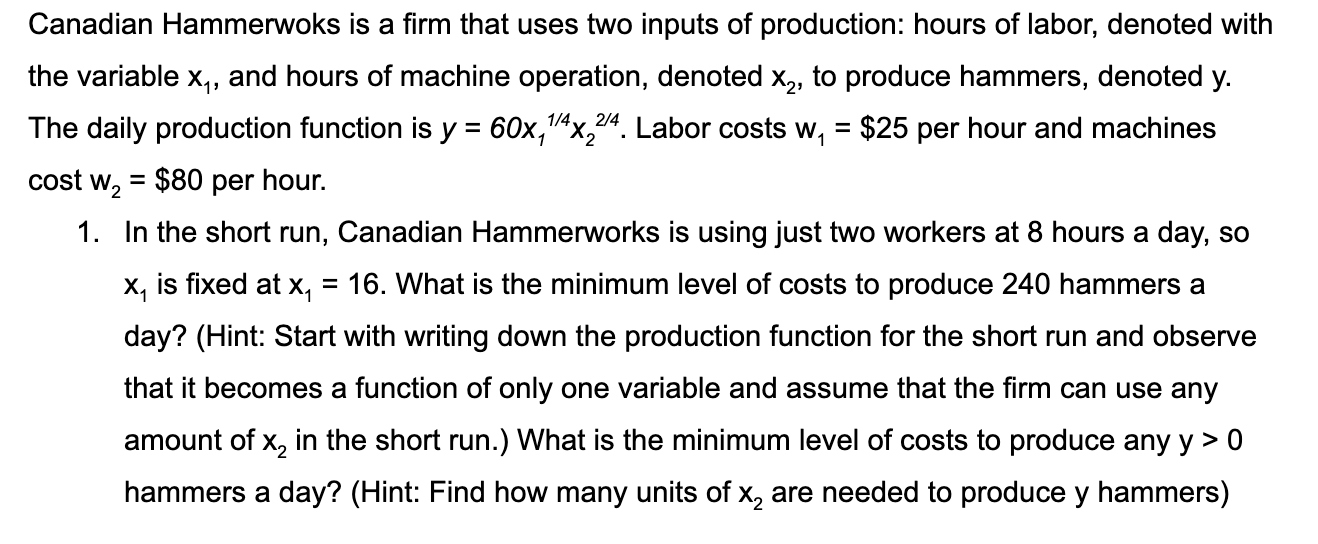### Create an Account

Home / Questions / Canadian Hammerwoks is a firm that uses two inputs of production hours of labor denoted ...

# Canadian Hammerwoks is a firm that uses two inputs of production hours of labor denoted with the variable

Hi, can you please give me a step by step solution for this problem?Canadian Hammerwoks is a firm that uses two inputs of production: hours of labor, denoted with the variable xq, and hours of machine operation, denoted X2, to produce hammers, denoted y. The daily production function is y = 60x14x22/4. Labor costs w, = \$25 per hour and machines cost wz = \$80 per hour. 1. In the short run, Canadian Hammerworks is using just two workers at 8 hours a day, so x, is fixed at x, = 16. What is the minimum level of costs to produce 240 hammers a day? (Hint: Start with writing down the production function for the short run and observe that it becomes a function of only one variable and assume that the firm can use any amount of x, in the short run.) What is the minimum level of costs to produce any y> 0 hammers a day? (Hint: Find how many units of x, are needed to produce y hammers)

Feb 07 2020 View more View LessGet Solution